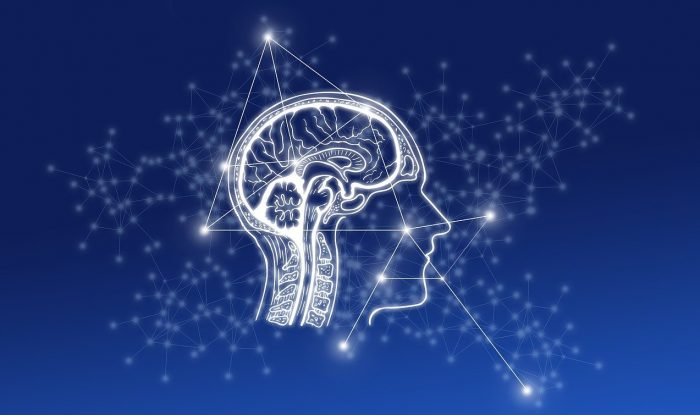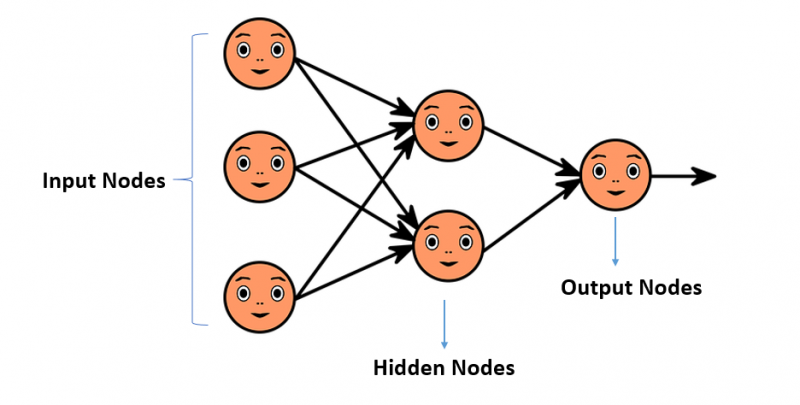## Introduction

Neural Network or popularly known as Artificial Neural Network (ANN), works similarly to the human brain’s neural network. It functions on the same principles as the nervous system works in our body. The neural network helps us to understand the relationships or patterns between complex data structures. There are different types of neural networks. Artificial Neural Network is widely used in Deep Learning Model. ANN and Deep Learning are augmented together to provide best-in-class solutions for various problems. Some of the applications are speech recognition, image compression, route detection, machine translation, forecasting, etc. Neural networks are also widely used in many business applications, such as marketing research, enterprise planning, fraud detection and risk assessment. Deep learning, a subset of Machine Learning, uses different types of neural networks to process data. The neural network works in hierarchical function and enables machines to process data with a non-parametric approach.## What is Artificial Neural Network (ANN)?

Artificial Neural Network is a series of computing algorithms that are designed to work like the human nervous system. It simulates the way human brain analyse and process information to the system. Similarly, how our brain can recognise pattern or trend, categorise information, etc., a neural network does the same. It has self-learning capabilities and gives best-in-class solutions to solve problems that would be difficult for a human to do. Artificial neural networks are widely used in Deep Learning Model which comes under the domain of Artificial Intelligence. Neural networks are widely used in financial operations, marketing research, enterprise planning, trading, business analytics, product maintenance and so forth.

## Neuron in Neural Network

Artificial Neural Network is based on the biological neural network, such as the brain. Just like a biological neural network, ANN is constantly self-learning based on observational data or training sets of data. A neuron, in a human brain, is the fundamental unit of the nervous system which communicate through both electrical and chemical signals. The human brain has more than 86 billion neurons. Each neuron is connected to n number of neurons, receives incoming signals (inputs) to reach the cell body (outputs). Artificial neuron (also called as Nodes) functions similar to biological neuron. The function used in a neuron is termed as an activation function. There are three types of neurons in neural network – input nodes, hidden nodes and output nodes. The input nodes take in information and presented as activation values, where each node is given a specific number. Then, it passed further into the network system, through hidden nodes, until it reaches the output nodes.### Major Types of Artificial Neural Networks

There are different types of artificial neural networks (ANN), which works with a different set of principles and based on their applicability. Each neural network system works similarly to our brain neural system. We can see applications of different types of neural networks in various Data Science Areas & Applications.

• Feedforward Neural Network – This type of neural network is the simplest form of ANN and considered as the most commonly used in today’s AI applications. In a feedforward neural network, data or the input passes in one direction. The input data passes through the different input nodes and exit on the output nodes. All the nodes are fully connected as every perceptron in one layer is connected with each node in the next layer. Hidden layers may or may not be present and further classified as a single-layered and multi-layered feedforward neural network. The single-layered feedforward neural network consists of only two layers of neurons and no hidden layers in between them. Whereas in multi-layered feedforward neural network, multiple hidden layers are present in between the input and output layers. The number of layers depends on the complexity of the function. Unlike other neural types, data or the input passes in one direction only and there is no backpropagation. Applications of feedforward neural networks are face recognition, speech recognition, object recognition, etc.
• Recurrent Neural Network – RNN involves the formation of loops, which means reoccurrence of operations or events. It works on the principle of loop formulation and output of a particular layer is saved and fed back to the input in predicting the outcome of the layer. Like feedforward neural network, the first layer is formed similarly with the product of the sum of the weights and the reoccurrence of operations or events process will start in subsequent layers, which means one step each to the next neuron. Recurrent neural network system work on the front propagation and each node will remember some information that it had in the previous time step. This makes each neuron act like a memory cell in performing computations. RNN has the ability to process inputs and share any outputs across time. This type of neural network is very effective in text prediction, language generation, speech recognition, etc. The recurrent neural network is much more complicated than the feedforward neural network.
• Convolutional Neural Network – This type of artificial neural network is commonly used for signal and image processing techniques. Convolutional neural network system performs the task with better accuracy and uses a three-dimensional arrangement of neurons instead of the standard two-dimensional array. The first layer in convolutional neural networks is called a convolutional layer. Convolutional neural network system consists of one or more convolutional layers and the propagation follows the unidirectional approach. These layers can either be fully interconnected or pooled. In the convolutional layer, each neuron only processes the information from a small part of the visual area. And the network can be much deeper but with much fewer parameters. Processing involves the image conversion from RGB scale to greyscale. Convolution neural networks are applied in techniques like semantic parsing, image recognition, video recognition, natural language processing, etc.
• Modular Neural Network – It consists of numerous different networks which totally works independently and these networks do not communicate with each other during the process. Each neural network works independently towards achieving the output. So basically, a modular neural network system comprises of more than one neural network system and follow the principle of “Divide & Conquer”. In a modular neural network system, large complex processes are breakdown into smaller independent components and decrease the problem complexity. Moreover, it speeds up the computational processes and has a broad range of applications.

### Applications of Neural Networks

• Fraud Detection
• Facial Recognition
• Speech Recognition
• Time Series Forecasting
• Classification & Regression
• Various Data Science Disciplines

### Advantages of Neural Networks

• The ability of parallel processing can perform more than one task at a time.
• There is no loss of data as it stored data in a network system instead of databases.
• The artificial neural network (ANN) has the ability of self-learning and fits a model which has a complex data structure.
• It has no restrictions on the data input and has the capability to handle high tolerance of messy data.

### Build your Neural Networks Models with Neural Designer

Neural Designer is a data science and machine learning platform that helps you build, train and deploy neural network models. Using the available data, you can simulate and optimise the processes at your organisation. Neural networks are the most powerful technique for machine learning. With Neural Designer, there is no need to write code or build block diagrams. Instead, the interface guides you through a sequence of well-defined steps. Neural Designer also supports the whole modelling cycle, from data preparation to model production.